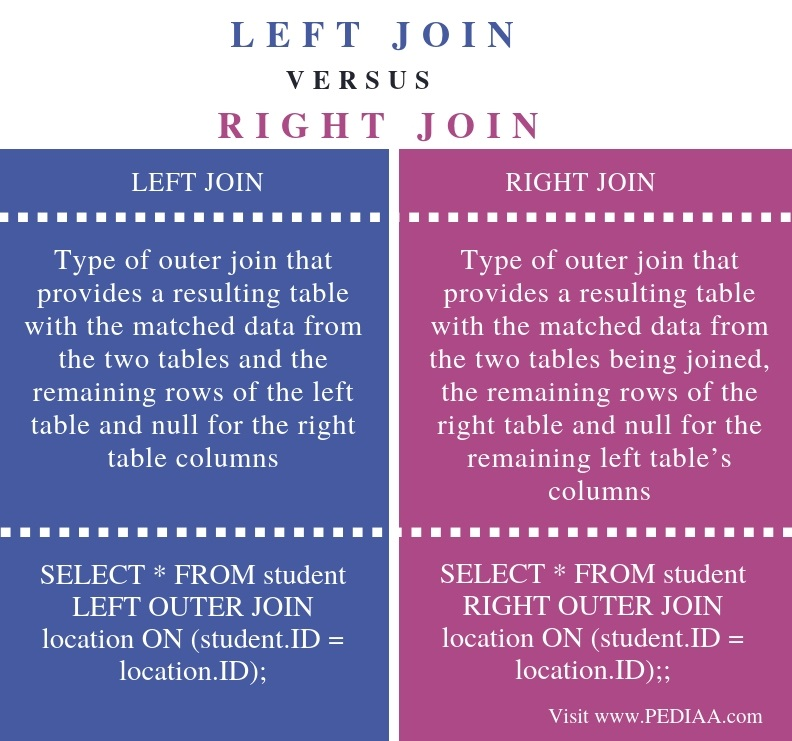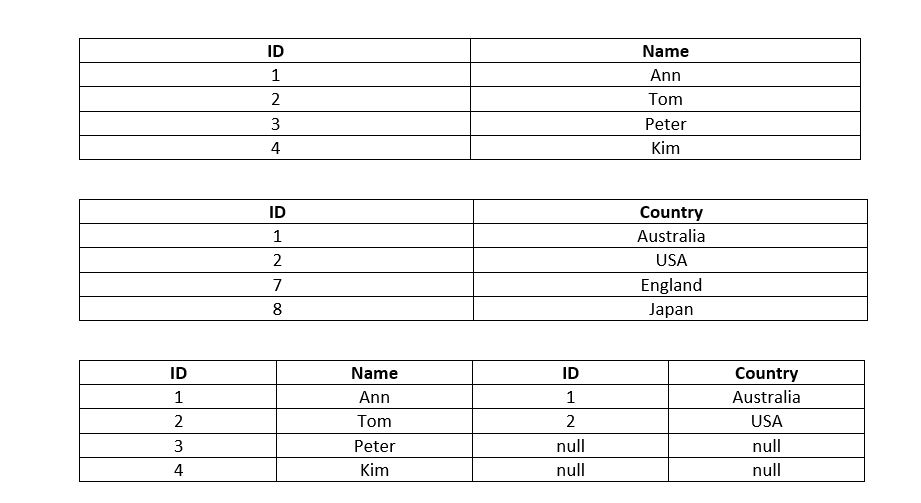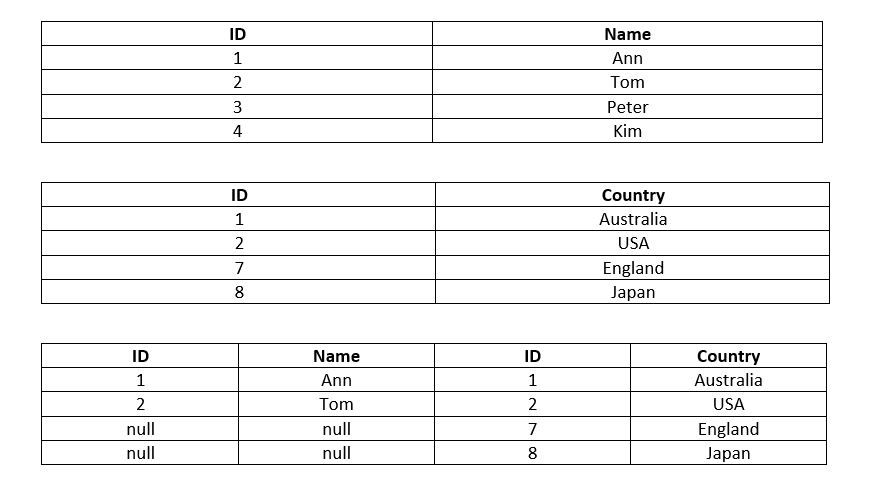# What is the Difference Between Left Join and Right Join

The main difference between left join and right join is that the left join provides the resulting table with the matched data from both tables and the remaining rows contain values from the left table and nulls from the right table. But, the right join provides the resulting table with matched data from both tables, and the remaining rows contain values from the right table and nulls from the left table.

Generally, DBMS is a software application that allows storing and managing data of the databases efficiently. A DBMS that is designed according to the relational model is called a Relational Database Management System (RDBMS). Structured Query Language (SQL) is the language that allows the programmer to write queries and execute them to perform operations on the data stored in the databases. Furthermore, there are multiple tables in a single database, and sometimes it is necessary to combine two tables. Joins is an alternative to this issue. Overall, there are various types of joins, and two of them are left join and right join. Both of them are types of outer joins.

### Key Areas Covered

1. What is Left Join
-Definition, Functionality
2. What is Right Join
-Definition, Functionality
3. Difference Between Left Join and Right Join
-Comparison of key differences

### Key Terms

Left Join, Right Join## What is Left Join

Left join returns a resulting table with the matched data from the two tables and the remaining rows of the left table and null from the right table columns. For example, refer to the below tables.The first table is the student table while the second table is the location table. Moreover, the third table is the table after performing the left join. The ID column is common to both tables.

The SQL statement for the left join is as follows.

SELECT * FROM student LEFT OUTER JOIN location ON (student.ID = location.ID);

In this join, the main concern is the left table, which is the student table. Therefore, the joined table has the values – ID and name – from the student table, and there are null values in the remaining fields of the right table columns.

Likewise, the left join table has the matching values and the records from the left table while including null for the values of the right table columns.

## What is Right Join

The right join returns a resulting table with the matched data of the two tables and remaining rows of the right table and null for the remaining left table’s columns.  For example, refer to the below tables.The first table is the student table while the second table is the location table. Moreover, the third table is the table after performing the right join. The ID column is common to both tables.

The SQL statement to perform this join is as follows.

SELECT * FROM student RIGHT OUTER JOIN location ON (student.ID = location.ID);

In this join, the main concern is the right table, which is the location table. Therefore, the joined table has the values – ID and country – from the location table, and there are null values in the remaining fields, which are from the left column.

Likewise, the right join table has the matching values and the records from the right table while including null for the values of the left table columns.

## Difference Between Left Join and Right Join

### Definition

Left Join is a type of outer join that provides a resulting table with the matched data from the two tables and the remaining rows of the left table and null from the right table columns. In contrast, the right join is a type of outer join that provides a resulting table with the matched data from the two tables being joined, the remaining rows of the right table and null for the remaining left table’s columns.

### Example SQL query

When the tables are student and location, the SQL statement for left join is SELECT * FROM student LEFT OUTER JOIN location ON (student.ID = location.ID); whereas, the SQL statement for right join is SELECT * FROM student RIGHT OUTER JOIN location ON (student.ID = location.ID);

### Conclusion

Sometimes, it is necessary to join two tables. Left join and right join are two types of joins. The main difference between left join and right join is that left join provides the resulting table with the matched data from both tables; remaining rows of the left table and assigns null to the right table columns. But, the right join provides the resulting table with matched data from both tables, remaining rows of the right table and assigns null to the left table columns. In brief, the programmer can perform left join or right join by writing the SQL statement.